## TENER: Adapting Transformer Encoder for Named Entity Recognition 笔记

article/2023/6/4 16:20:41

### TENER: Adapting Transformer Encoder for Named Entity Recognition

• Abstract（摘要）
• 1 Introduction（介绍）
• 2 Related Work（相关工作）
• 2.1 Neural Architecture for NER（NER 的神经网络架构）
• 2.2 Transformer
• 2.2.1 Transformer Encoder Architecture
• 2.2.2 Position Embedding
• 3 Proposed Model（目标模型）
• 3.1 Embedding Layer
• 3.2 Encoding Layer with Adapted Transformer
• 3.2.1 Direction- and Distance-Aware Attention
• 3.2.2 Un-scaled Dot-Product Attention
• 3.3 CRF Layer
• Experiment（实验）
• 4.1 Data
• Conclusion
• TENER运行流程
• 整体流程
• TENER模型训练流程
• Transformer编码器网络结构

## Abstract（摘要）

Bidirectional long short-term memory networks (BiLSTMs) have been widely used as an encoder for named entity recognition (NER) task. Recently, the fully-connected self-attention architecture (aka Transformer) is broadly adopted in various natural language processing (NLP) tasks owing to its parallelism and advantage in modeling the longrange context. Nevertheless, the performance of the vanilla Transformer in NER is not as good as it is in other NLP tasks. In this paper, we propose TENER, a NER architecture adopting adapted Transformer Encoder to model the character-level features and wordlevel features.

By incorporating the directionaware, distance-aware and un-scaled attention, we prove the Transformer-like encoder is just as effective for NER as other NLP tasks.

Experiments on six NER datasets show that TENER achieves superior performance than the prevailing BiLSTM-based models.

## 1 Introduction（介绍）

The named entity recognition (NER) is the task of finding the start and end of an entity in a sentence and assigning a class for this entity. NER has been widely studied in the field of natural language processing (NLP) because of its potential assistance in question generation (Zhou et al., 2017), relation extraction (Miwa and Bansal, 2016), and coreference resolution (Fragkou, 2017). Since (Collobert et al., 2011), various neural models have been introduced to avoid hand-crafted features (Huang et al., 2015; Ma and Hovy, 2016; Lample et al., 2016).

NER is usually viewed as a sequence labeling task, the neural models usually contain three components: word embedding layer, context encoder layer, and decoder layer (Huang et al., 2015; Ma and Hovy, 2016; Lample et al., 2016; Chiu and Nichols, 2016; Chen et al., 2019; Zhang et al., 2018; Gui et al., 2019b). The difference between various NER models mainly lies in the variance in these components.
NER 通常被视为序列标注任务，神经模型通常包含三个组件：词嵌入层、上下文编码器层和解码器层（Huang et al., 2015; Ma and Hovy, 2016; Lample et al., 2016; Chiu and Nichols, 2016; Chen et al., 2019; Zhang et al., 2018; Gui et al., 2019b)。各种NER模型之间的差异主要在于它们所用组件的差异。

Recurrent Neural Networks (RNNs) are widely employed in NLP tasks due to its sequential characteristic, which is aligned well with language. Specifically, bidirectional long short-term memory networks (BiLSTM) (Hochreiter and Schmidhuber, 1997) is one of the most widely used RNN structures. (Huang et al., 2015) was the first one to apply the BiLSTM and Conditional Random Fields (CRF) (Lafferty et al., 2001) to sequence labeling tasks. Owing to BiLSTM’s high power to learn the contextual representation of words, it has been adopted by the majority of NER models as the encoder (Ma and Hovy, 2016; Lample et al., 2016; Zhang et al., 2018; Gui et al., 2019b).

Recently, Transformer (Vaswani et al., 2017) began to prevail in various NLP tasks, like machine translation (Vaswani et al., 2017), language modeling (Radford et al., 2018), and pretraining models (Devlin et al., 2018). The Transformer encoder adopts a fully-connected self-attention structure to model the long-range context, which is the weakness of RNNs. Moreover, Transformer has better parallelism ability than RNNs. However, in the NER task, Transformer encoder has been reported to perform poorly (Guo et al., 2019), our experiments also confirm this result. Therefore, it is intriguing to explore the reason why Transformer does not work well in NER task.

In this paper, we analyze the properties of Transformer and propose two specific improvements for NER.

The first is that the sinusoidal position embedding used in the vanilla Transformer is aware of distance but unaware of the directionality. In addition, this property will lose when used in the vanilla Transformer. However, both the direction and distance information are important in the NER task. For example in Fig 1, words after “in” are more likely to be a location or time than words before it, and words before “Inc.” are mostly likely to be of the entity type “ORG”. Besides, an entity is a continuous span of words. Therefore, the awareness of distance might help the word better recognizes its neighbor. To endow the Transformer with the ability of direction- and distanceawareness, we adopt the relative positional encoding (Shaw et al., 2018; Huang et al., 2019; Dai et al., 2019). instead of the absolute position encoding. We propose a revised relative positional encoding that uses fewer parameters and performs better.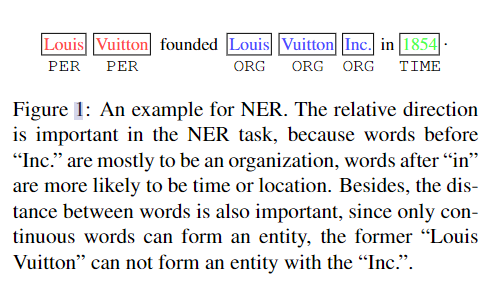The second is an empirical finding. The attention distribution of the vanilla Transformer is scaled and smooth. But for NER, a sparse attention is suitable since not all words are necessary to be attended. Given a current word, a few contextual words are enough to judge its label. The smooth attention could include some noisy information. Therefore, we abandon the scale factor of dot-production attention and use an unscaled and sharp attention.

With the above improvements, we can greatly boost the performance of Transformer encoder for NER.

Other than only using Transformer to model the word-level context, we also tried to apply it as a character encoder to model word representation with character-level information. The previous work has proved that character encoder is necessary to capture the character-level features and alleviate the out-of-vocabulary (OOV) problem (Lample et al., 2016; Ma and Hovy, 2016; Chiu and Nichols, 2016; Xin et al., 2018). In NER, CNN is commonly used as the character encoder. However, we argue that CNN is also not perfect for representing character-level information, because the receptive field of CNN is limited, and the kernel size of the CNN character encoder is usually 3, which means it cannot correctly recognize 2-gram or 4-gram patterns. Although we can deliberately design different kernels, CNN still cannot solve patterns with discontinuous characters, such as “un…ily” in “unhappily” and “unnecessarily”. Instead, the Transformer-based character encoder shall not only fully make use of the concurrence power of GPUs, but also have the potentiality to recognize different n-grams and even discontinuous patterns. Therefore, in this paper, we also try to use Transformer as the character encoder, and we compare four kinds of character encoders.

N-gram 模式，当前词只与它前面n − 1个词相关。

(1) 计算复杂度：n越大，计算复杂度越大（指数级增长）。
(2) 模型效果：理论上n越大越好，但n越大，模型效果的提升幅度越小。

In summary, to improve the performance of the Transformer-based model in the NER task, we explicitly utilize the directional relative positional encoding, reduce the number of parameters and sharp the attention distribution. After the adaptation, the performance raises a lot, making our model even performs better than BiLSTM based models. Furthermore, in the six NER datasets, we achieve state-of-the-art performance among models without considering the pre-trained language models or designed features.

## 2.1 Neural Architecture for NER（NER 的神经网络架构）

Collobert et al. (2011) utilized the Multi-Layer Perceptron (MLP) and CNN to avoid using taskspecific features to tackle different sequence labeling tasks, such as Chunking, Part-of-Speech (POS) and NER. In (Huang et al., 2015), BiLSTM-CRF was introduced to solve sequence labeling questions. Since then, the BiLSTM has been extensively used in the field of NER (Chiu and Nichols, 2016; Dong et al., 2016; Yang et al., 2018; Ma and Hovy, 2016).
(Collobert et al，2011) 利用多层感知器 (MLP) 和 CNN 来避免使用特定于任务的特征来处理不同的序列标记任务，例如分块、词性 (POS) 和 NER。在 (Huang et al., 2015) 中，引入了 BiLSTM-CRF 来解决序列标记问题。从那时起，BiLSTM 已广泛用于 NER 领域（Chiu 和 Nichols，2016；Dong 等人，2016；Yang 等人，2018；Ma 和 Hovy，2016）。

Despite BiLSTM’s great success in the NER task, it has to compute token representations one by one, which massively hinders full exploitation of GPU’s parallelism. Therefore, CNN has been proposed by (Strubell et al., 2017; Gui et al., 2019a) to encode words concurrently. In order to enlarge the receptive field of CNNs, (Strubell et al., 2017) used iterative dilated CNNs (IDCNN).

Since the word shape information, such as the capitalization and n-gram, is important in recognizing named entities, CNN and BiLSTM have been used to extract character-level information (Chiu and Nichols, 2016; Lample et al., 2016; Ma and Hovy, 2016; Strubell et al., 2017; Chen et al., 2019).

Almost all neural-based NER models used pretrained word embeddings, like Word2vec and Glove (Pennington et al., 2014; Mikolov et al., 2013). And when contextual word embeddings are combined, the performance of NER models will boost a lot (Peters et al., 2017, 2018; Akbik et al., 2018). ELMo introduced by (Peters et al., 2018) used the CNN character encoder and BiLSTM language models to get contextualized word representations. Except for the BiLSTM based pre-trained models, BERT was based on Transformer (Devlin et al., 2018).

## 2.2 Transformer

Transformer was introduced by (Vaswani et al., 2017), which was mainly based on self-attention. It achieved great success in various NLP tasks. Since the self-attention mechanism used in the Transformer is unaware of positions, to avoid this shortage, position embeddings were used (Vaswani et al., 2017; Devlin et al., 2018). Instead of using the sinusoidal position embedding (Vaswani et al., 2017) and learned absolute position embedding, Shaw et al. (2018) argued that the distance between two tokens should be considered when calculating their attention score. Huang et al. (2019) reduced the computation complexity of relative positional encoding from O(l2d) toO(ld), where l is the length of sequences and d is the hidden size. Dai et al. (2019) derived a new form of relative positional encodings, so that the relative relation could be better considered.
Transformer 是由 (Vaswani et al., 2017) 引入的，主要基于 self-attention。它在各种 NLP 任务中取得了巨大的成功。由于 Transformer 中使用的自注意力机制不知道位置，为了避免这种短缺，使用了位置嵌入（Vaswani et al., 2017; Devlin et al., 2018）。（Shaw et al., 2018）没有使用正弦位置嵌入（Vaswani et al., 2017）和学习绝对位置嵌入。（Shaw et al., 2018）认为在计算它们的注意力分数时应该考虑两个标记之间的距离。（Huang et al., 2019）将相对位置编码的计算复杂度从 O(l2d) 降低到 O(ld)，其中 l 是序列的长度，d 是隐藏大小。（Dai et al., 2019）推导出一种新形式的相对位置编码，以便更好地考虑相对关系。

## 2.2.1 Transformer Encoder Architecture

We first introduce the Transformer encoder proposed in (Vaswani et al., 2017). The Transformer encoder takes in an matrix H ∈ Rl×d, where l the sequence length, d is the input dimension. Then three learnable matrix Wq, Wk, Wv are used to project H into different spaces. Usually, the matrix size of the three matrix are all Rd×dk , where dk is a hyper-parameter. After that, the scaled dotproduct attention can be calculated by the following equations,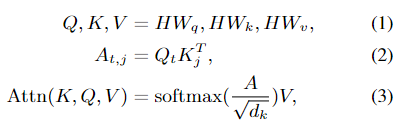where Qt is the query vector of the tth token, j is the token the tth token attends. Kj is the key vector representation of the jth token. The softmax is along the last dimension. Instead of using one group of Wq, Wk, Wv, using several groups will enhance the ability of self-attention. When several groups are used, it is called multi-head selfattention, the calculation can be formulated as follows,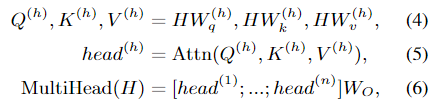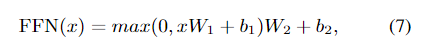where n is the number of heads, the superscript h represents the head index. [head(1); …; head(n)]means concatenation in the last dimension. Usually dk × n = d, which means the output of [head(1); …; head(n)] will be of size Rl×d. Wo is a learnable parameter, which is of size Rd×d.

The output of the multi-head attention will be further processed by the position-wise feedforward networks, which can be represented as follows,

where W1, W2, b1, b2 are learnable parameters, and W1 ∈ Rd×dff , W2 ∈ Rdff×d, b1 ∈ Rdff ,b2 ∈ Rd. dff is a hyper-parameter. Other components of the Transformer encoder includes layer normalization and Residual connection, we use them the same as (Vaswani et al., 2017).

## 2.2.2 Position Embedding

The self-attention is not aware of the positions of different tokens, making it unable to capture the sequential characteristic of languages. In order to solve this problem, (Vaswani et al., 2017) suggested to use position embeddings generated by sinusoids of varying frequency. The tth token’s position embedding can be represented by the following equations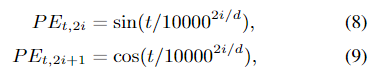self-attention 不知道不同标记的位置，使其无法捕捉语言的顺序特征。为了解决这个问题，(Vaswani et al., 2017) 建议使用由不同频率的正弦曲线生成的位置嵌入。第 t 个 token 的位置嵌入可以用以下等式表示

where i is in the range of [0, d/2 ], d is the input dimension. This sinusoid based position embedding makes Transformer have an ability to model the position of a token and the distance of each two tokens. For any fixed offset k, PEt+k can be represented by a linear transformation of PEt (Vaswani et al., 2017).

## 3 Proposed Model（目标模型）

In this paper, we utilize the Transformer encoder to model the long-range and complicated interactions of sentence for NER. The structure of proposed model is shown in Fig 2. We detail each parts in the following sections.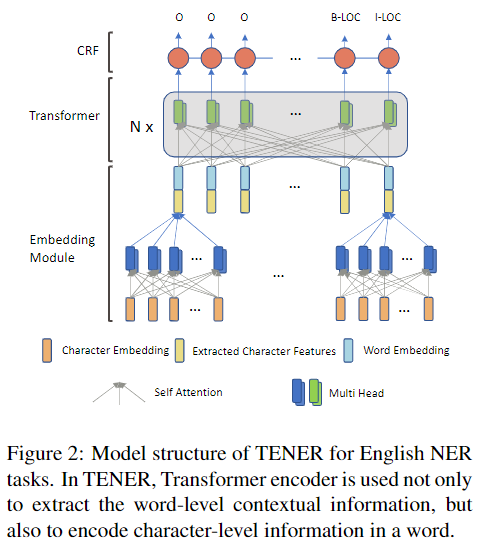## 3.1 Embedding Layer

To alleviate the problems of data sparsity and outof-vocabulary (OOV), most NER models adopted the CNN character encoder (Ma and Hovy, 2016; Ye and Ling, 2018; Chen et al., 2019) to represent words. Compared to BiLSTM based character encoder (Lample et al., 2016; Ghaddar and Langlais, 2018), CNN is more efficient. Since Transformer can also fully exploit the GPU’s parallelism, it is interesting to use Transformer as the character encoder. A potential benefit of Transformer-based character encoder is to extract different n-grams and even uncontinuous character patterns, like “un…ily” in “unhappily” and “uneasily”. For the model’s uniformity, we use the “adapted Transformer” to represent the Transformer introduced in next subsection.

The final word embedding is the concatenation of the character features extracted by the character encoder and the pre-trained word embeddings.

# @cache_results 作为修饰器帮用户将处理后的数据保存到本地文件。
# 第二次运行时，如果设置的 _refresh 是为 False 和_cachefp 不为 None，则会去本地读取数据返回，不需要重新执行 load_data() 函数。
@cache_results(name, _refresh=False)
def load_data():# 替换路径if dataset == 'conll2003':# conll2003的lr不能超过0.002paths = {'test': "./datasets/conll2003/eng.testa",'train': "./datasets/conll2003/eng.train",'dev': "./datasets/conll2003/eng.testb"}data = Conll2003NERPipe(encoding_type=encoding_type).process_from_file(paths)elif dataset == 'en-ontonotes':# 会使用这个文件夹下的train.txt, test.txt, dev.txt等文件paths = './datasets/en-ontonotes/english'data = OntoNotesNERPipe(encoding_type=encoding_type).process_from_file(paths)char_embed = None# char representation 由 char embedding 与对应的 word embedding concat 起来得到# char embedding 是通过将单词的字符当做一个序列，经过 cnn 或者 rnn 以后得到的，然后# 这里设置三种字符嵌入类型，这里同论文 3.1 采用CNN 字符编码器if char_type == 'cnn':char_embed = CNNCharEmbedding(vocab=data.get_vocab('words'), embed_size=30, char_emb_size=30, filter_nums=,kernel_sizes=, word_dropout=0, dropout=0.3, pool_method='max',include_word_start_end=False, min_char_freq=2)elif char_type in ['adatrans', 'naive']:char_embed = TransformerCharEmbed(vocab=data.get_vocab('words'), embed_size=30, char_emb_size=30,word_dropout=0,dropout=0.3, pool_method='max', activation='relu',min_char_freq=2, requires_grad=True, include_word_start_end=False,char_attn_type=char_type, char_n_head=3, char_dim_ffn=60,char_scale=char_type == 'naive',char_dropout=0.15, char_after_norm=True)elif char_type == 'lstm':char_embed = LSTMCharEmbedding(vocab=data.get_vocab('words'), embed_size=30, char_emb_size=30, word_dropout=0,dropout=0.3, hidden_size=100, pool_method='max', activation='relu',min_char_freq=2, bidirectional=True, requires_grad=True,include_word_start_end=False)word_embed = StaticEmbedding(vocab=data.get_vocab('words'),model_dir_or_name='en-glove-6b-100d',requires_grad=True, lower=True, word_dropout=0, dropout=0.5,only_norm_found_vector=normalize_embed)if char_embed is not None:embed = StackEmbedding([word_embed, char_embed], dropout=0, word_dropout=0.02)else:word_embed.word_drop = 0.02embed = word_embeddata.rename_field('words', 'chars')return data, embed


## 3.2 Encoding Layer with Adapted Transformer

Although Transformer encoder has potential advantage in modeling long-range context, it is not working well for NER task. In this paper, we propose an adapted Transformer for NER task with two improvements.

### 3.2.1 Direction- and Distance-Aware Attention

Inspired by the success of BiLSTM in NER tasks, we consider what properties the Transformer lacks compared to BiLSTM-based models. One observation is that BiLSTM can discriminatively collect the context information of a token from its left and right sides. But it is not easy for the Transformer to distinguish which side the context information comes from.

Although the dot product between two sinusoidal position embeddings is able to reflect their distance, it lacks directionality and this property will be broken by the vanilla Transformer attention. To illustrate this, we first prove two properties of the sinusoidal position embeddings.

Property 1. For an offset k and a position t,PETt+k PEt only depends on k, which means the dot product of two sinusoidal position embeddings can reflect the distance between two tokens.

Proof. Based on the definitions of Eq.(8) and Eq.(9), the position embedding of t-th token is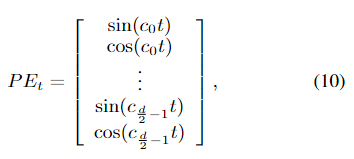where d is the dimension of the position embedding, ci is a constant decided by i, and its value is 1/100002i/d.

Therefore,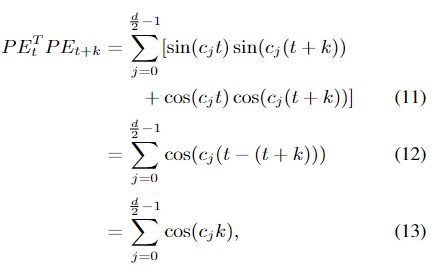where Eq.(11) to Eq.(12) is based on the equationcos(x − y) = sin(x) sin(y) + cos(x) cos(y).

Property 2. For an offset k and a position t, PETt PEt−k = PETt PEt+k, which means the sinusoidal position embeddings is unware of directionality.

Proof. Let j = t − k, according to property 1, we have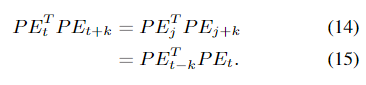The relation between d, k and PETt PEt+k is displayed in Fig 3. The sinusoidal position embeddings are distance-aware but lacks directionality.
d, k 和 PETt PEt+k 之间的关系如图 3 所示。正弦位置嵌入是距离感知的，但缺乏方向性。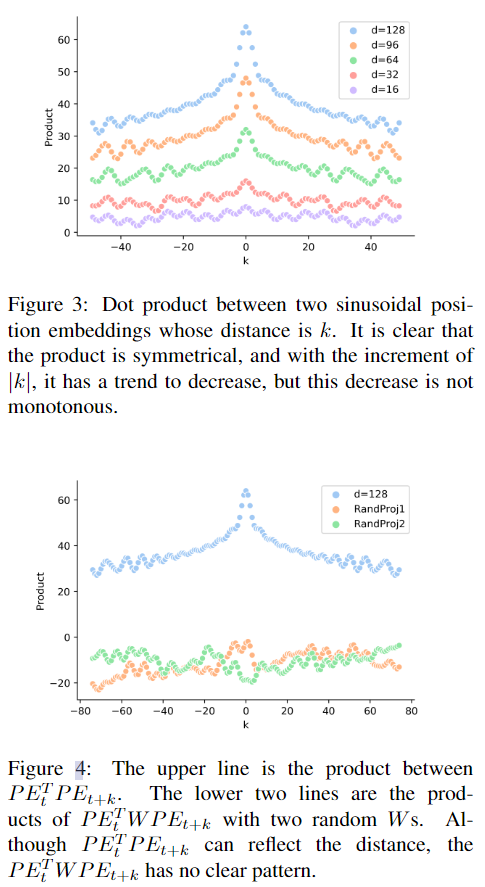However, the property of distance-awareness also disappears when PEt is projected into the query and key space of self-attention. Since in vanilla Transformer the calculation between PEt and PEt+k is actually PETt WqT Wk PEt+k, where Wq, Wk are parameters in Eq.(1). Mathematically, it can be viewed as PETt W PEt+k with only one parameter W. The relation between PETt PEt+k and PETt W PEt+k is depicted in Fig 4.

Therefore, to improve the Transformer with direction- and distance-aware characteristic, we calculate the attention scores using the equations below: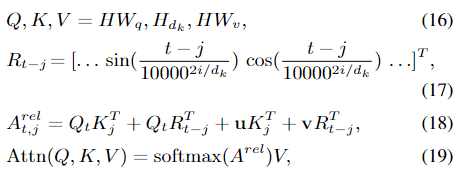where t is index of the target token, j is the index of the context token, Qt, Kj is the query vector and key vector of token t, j respectively,Wq, Wv ∈ Rd×dk . To get Hdk ∈ Rl×dk , we first split H into d/dk partitions in the second dimension, then for each head we use one partition. u ∈ Rdk , v ∈ Rdk are learnable parameters, Rt−j is the relative positional encoding, andRt−j ∈ Rdk , i in Eq.(17) is in the range [0, dk2 ].QT t Kj in Eq.(18) is the attention score between two tokens; QT t Rt−j is the tth token’s bias on certain relative distance; uT Kj is the bias on the jth token; vT Rt−j is the bias term for certain distance and direction.

    def forward(self, x, mask):""":param x: batch_size x max_len x d_model:param mask: batch_size x max_len:return:"""batch_size, max_len, d_model = x.size()pos_embed = self.pos_embed(mask)  # l x head_dimqkv = self.qkv_linear(x)  # batch_size x max_len x d_model3q, k, v = torch.chunk(qkv, chunks=3, dim=-1)q = q.view(batch_size, max_len, self.n_head, -1).transpose(1, 2)k = k.view(batch_size, max_len, self.n_head, -1).transpose(1, 2)v = v.view(batch_size, max_len, self.n_head, -1).transpose(1, 2)  # b x n x l x drw_head_q = q + self.r_r_bias[:, None]# 一种非常方便的多维矩阵运算AC = torch.einsum('bnqd,bnkd->bnqk', [rw_head_q, k])  # b x n x l x d, n是headD_ = torch.einsum('nd,ld->nl', self.r_w_bias, pos_embed)[None, :, None]  # head x 2max_len, 每个head对位置的biasB_ = torch.einsum('bnqd,ld->bnql', q, pos_embed)  # bsz x head  x max_len x 2max_len，每个query对每个shift的偏移E_ = torch.einsum('bnqd,ld->bnql', k, pos_embed)  # bsz x head x max_len x 2max_len, key对relative的biasBD = B_ + D_  # bsz x head x max_len x 2max_len, 要转换为bsz x head x max_len x max_lenBDE = self._shift(BD) + self._transpose_shift(E_)attn = AC + BDEattn = attn / self.scaleattn = attn.masked_fill(mask[:, None, None, :].eq(0), float('-inf'))attn = F.softmax(attn, dim=-1)attn = self.dropout_layer(attn)v = torch.matmul(attn, v).transpose(1, 2).reshape(batch_size, max_len, d_model)  # b x n x l x dreturn v


Based on Eq.(17), we have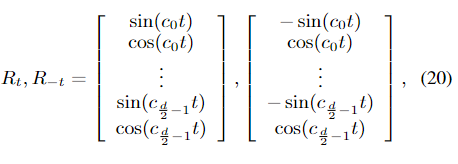because sin(−x) = − sin(x), cos(x) = cos(−x). This means for an offset t, the forward and backward relative positional encoding are the same with respect to the cos(cit) terms, but is the opposite with respect to the sin(cit) terms. Therefore, by using Rt−j , the attention score can distinguish different directions and distances.

The above improvement is based on the work (Shaw et al., 2018; Dai et al., 2019). Since the size of NER datasets is usually small, we avoid direct multiplication of two learnable parameters, because they can be represented by one learnable parameter. Therefore we do not use Wk in Eq.(16). The multi-head version is the same as Eq.(6), but we discard Wo since it is directly multiplied by W1in Eq.(7).

## 3.2.2 Un-scaled Dot-Product Attention

The vanilla Transformer use the scaled dotproduct attention to smooth the output of softmax function. In Eq.(3), the dot product of key and value matrices is divided by the scaling factor dk\sqrt{dk}.

We empirically found that models perform better without the scaling factor dk\sqrt{dk}. We presume this is because without the scaling factor the attention will be sharper. And the sharper attention might be beneficial in the NER task since only few words in the sentence are named entities.

## 3.3 CRF Layer

In order to take advantage of dependency between different tags, the Conditional Random Field (CRF) was used in all of our models. Given a sequence s = [s1, s2, …, sT], the corresponding golden label sequence is y = [y1, y2, …, yT], and Y(s) represents all valid label sequences. The probability of y is calculated by the following equation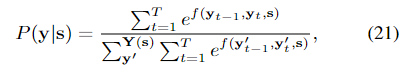where f (yt−1, yt, s) computes the transition score from yt−1 to yt and the score for yt. The optimization target is to maximize P(y|s). When decoding, the Viterbi Algorithm is used to find the path achieves the maximum probability.

# 条件随机场 tag_vocab：标签的数量，include_start_end_trans：是否考虑各个tag作为开始以及结尾的分数
self.crf = ConditionalRandomField(len(tag_vocab), include_start_end_trans=True, allowed_transitions=trans)


## 4.1 Data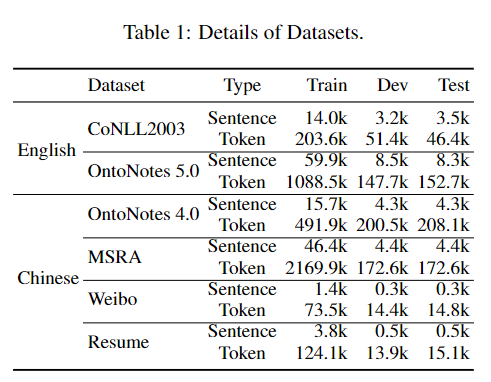We evaluate our model in two English NER datasets and four Chinese NER datasets.
(1) CoNLL2003 is one of the most evaluated English NER datasets, which contains four different named entities: PERSON, LOCATION, ORGANIZATION, and MISC (Sang and Meulder, 2003).
(2) OntoNotes 5.0 is an English NER dataset whose corpus comes from different domains, such as telephone conversation, newswire. We exclude the New Testaments portion since there is no named entity in it (Chen et al., 2019; Chiu and Nichols, 2016). This dataset has eleven entity names and seven value types, like CARDINAL, MONEY, LOC.
(3) Weischedel (2011) released OntoNotes 4.0. In this paper, we use the Chinese part. We adopted the same pre-process as (Che et al., 2013).
(4) The corpus of the Chinese NER dataset MSRA came from news domain (Levow, 2006).
(5) Weibo NER was built based on text in Chinese social media Sina Weibo (Peng and Dredze, 2015), and it contained 4 kinds of entities.
(6) Resume NER was annotated by (Zhang and Yang, 2018).

（1）CoNLL2003 是评估最多的英语 NER 数据集之一，它包含四个不同的命名实体：PERSON、LOCATION、ORGANIZATION 和 MISC (Sang and Meulder, 2003)。
（2）OntoNotes 5.0是一个英文NER数据集，其语料来自不同领域，如电话交谈、新闻专线。我们排除了新约部分，因为其中没有命名实体（hen et al., 2019; Chiu and Nichols, 2016）。该数据集有 11 个实体名称和 7 种值类型，如 CARDINAL、MONEY、LOC。
（3）Weischedel (2011) 发布了 OntoNotes 4.0。在本文中，我们使用中文部分。我们采用了与 (Che et al., 2013) 相同的预处理过程。
（4）中文 NER 数据集MSRA的语料来自新闻领域（Levow，2006）。
（5）微博 NER 是基于中国社交媒体新浪微博中的文本构建的（Peng and Dredze, 2015），它包含 4 种实体。
（6）NER 由 (Zhang and Yang, 2018) 重新注释。

Their statistics are listed in Table 1. For all datasets, we replace all digits with “0”, and use the BIOES tag schema. For English, we use the Glove 100d pre-trained embedding (Pennington et al., 2014). For the character encoder, we use 30d randomly initialized character embeddings. More details on models’ hyper-parameters can be found in the supplementary material. For Chinese, we used the character embedding and bigram embedding released by (Zhang and Yang, 2018). All pretrained embeddings are finetuned during training. In order to reduce the impact of randomness, we ran all of our experiments at least three times, and its average F1 score and standard deviation are reported.

We used random-search to find the optimal hyper-parameters, hyper-parameters and their ranges are displayed in the supplemental material. We use SGD and 0.9 momentum to optimize the model. We run 100 epochs and each batch has 16 samples. During the optimization, we use the triangle learning rate (Smith, 2017) where the learning rate rises to the pre-set learning rate at the first 1% steps and decreases to 0 in the left 99% steps. The model achieves the highest development performance was used to evaluate the test set. The hyper-parameter search range and other settings can be found in the supplementary material. Codes are available at https://github.com/fastnlp/TENER.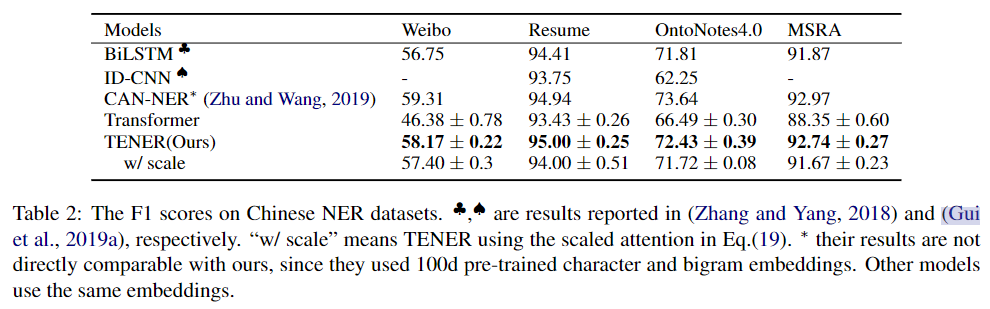## Conclusion

In this paper, we propose TENER, a model adopting Transformer Encoder with specific customizations for the NER task. Transformer Encoder has a powerful ability to capture the long-range context. In order to make the Transformer more suitable to the NER task, we introduce the direction-aware, distance-aware and un-scaled attention. Experiments in two English NER tasks and four Chinese NER tasks show that the performance can be massively increased. Under the same pretrained embeddings and external knowledge, our proposed modification outperforms previous models in the six datasets. Meanwhile, we also found the adapted Transformer is suitable for being used as the English character encoder, because it has the potentiality to extract intricate patterns from characters. Experiments in two English NER datasets show that the adapted Transformer character encoder performs better than BiLSTM and CNN character encoders.

## TENER模型训练流程

TENER模型->进入输入全连接层->进入Transformer编码器->进入Dropout层->进入输出全连接层->进行log_softmax归一化->返回loss

### Transformer编码器网络结构

num_layers个编码器层（即进行num_layers次迭代）：

http://www.ngui.cc/article/show-1007579.html

### 实验十 图着色问题

《算法设计与分析》实验报告 所在院系 计算机与信息工程学院 学生学号 学生姓名 年级专业 2020级计算机科学与技术 授课教师 彭绪富 学 期 2022-2023学年第一学期 提交时间 2022年11月16日 目录 实验十-1&#xff1a;图着色问题 一、实验目的…

### pip安装及国内源更换

# pip安装 python3&#xff1a; curl https://bootstrap.pypa.io/get-pip.py -o get-pip.py python3 get-pip.py python2: curl https://bootstrap.pypa.io/pip/2.7/get-pip.py -o get-pip2.py python2 get-pip2.py # pip国内的一些镜像 阿里云 http://mirrors.aliyu…

### vue2 和vue3动态绑定src

vue2&#xff1a; webpack 创建的vue2可以通过 require对图片进行动态绑定 <script> export default{data(){return{list:[{id:1,img:require("./assets/logo.png")},{id:2,img:require("./assets/logo.png")},{id:3,img:require("./assets/l…

### TryHackMe-Sustah（boot2root）

Sustah 开发人员在他们的游戏中添加了反作弊措施。你是 能否突破限制以访问其内部 CMS&#xff1f; 端口扫描 循例 nmap Web枚举 80端口没啥东西&#xff0c;看一下8085端口 gobuster扫一下 /ping似乎没什么东西 回来home&#xff0c;看看burp 使用bash生成数字字典 使用ff…